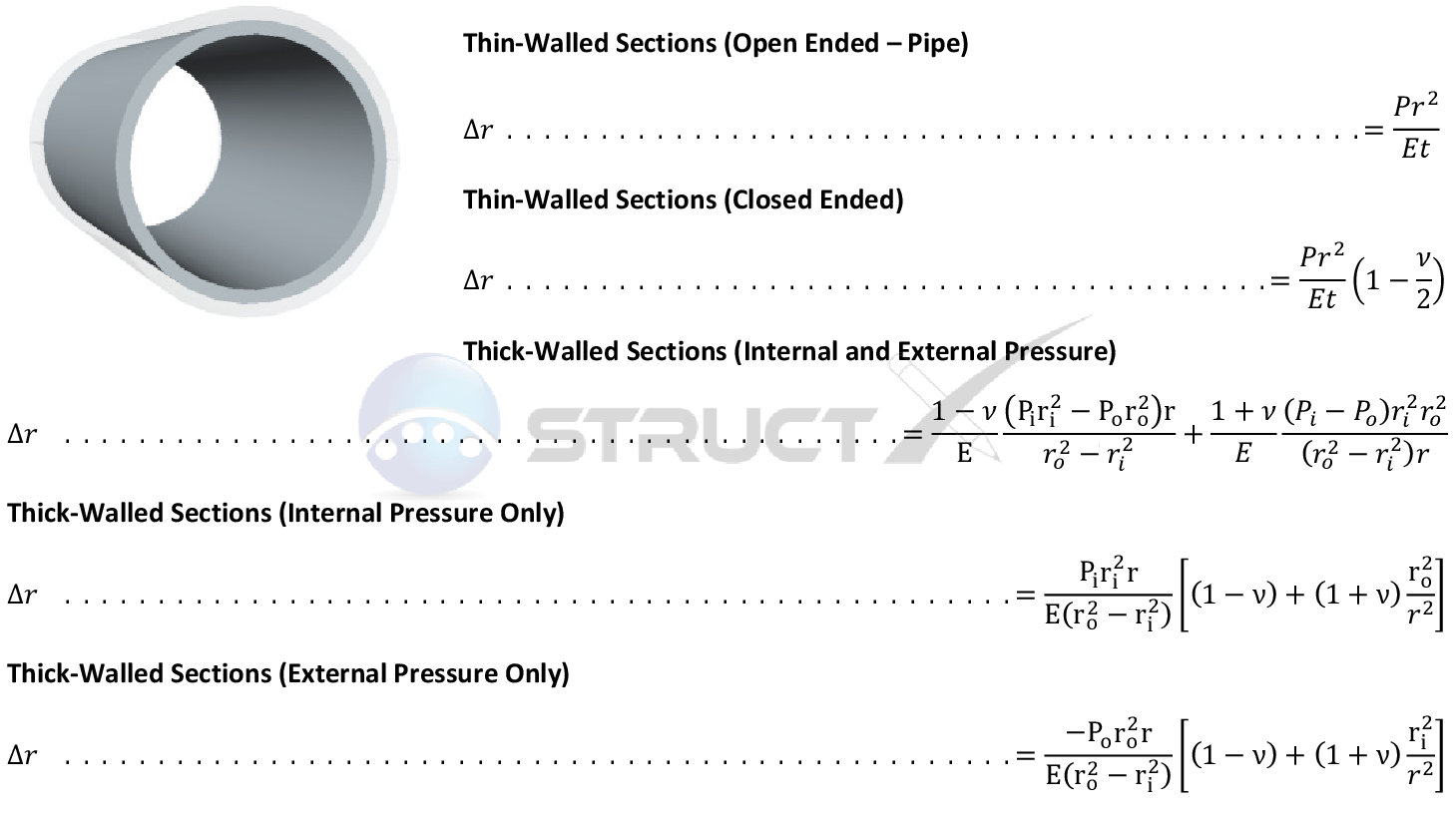# Pressure Vessel - Radial DisplacementMore Cases# Notation and Units

## Metric and Imperial Units

The above formulas may be used with both imperial and metric units. As with all calculations care must be taken to keep consistent units throughout with examples of units which should be adopted listed below:

# Notation

• ∆r = change in radius, in or mm
• E = modulus of elasticity, psi or MPa
• P = pressure under consideration, psi or MPa
• Pi = internal pressure, psi or MPa
• Po = external pressure, psi or MPa
• r = radius to point of of interest, in or mm
• ri = internal radius, in or mm
• ro = external radius, in or mm
• t = wall thickness, in or mm
• v = Poisson's ratio, unitless